# Inductor

Inductor is an electrical component that stores energy in magnetic field.

The inductor is made of a coil of conducting wire.

In an electrical circuit schematics, the inductor marked with the letter L.

The inductance is measured in units of Henry [L].

Inductor reduce current in AC circuits and short circuit in DC circuits.

### Inductor picture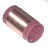### Inductor symbols

##### Inductor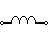##### Iron core inductor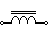##### Variable inductor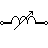### Inductors in series

For several inductors in series the total equivalent inductance is:

LTotal = L1+L2+L3+...

### Inductors in parallel

For several inductors in parallel the total equivalent inductance is: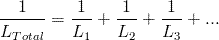### Inductor's voltage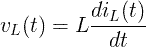### Inductor's current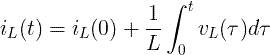### Energy of inductor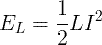XL = ωL

Cartesian form:

ZL = jXL = jωL

Polar form:

ZL = XL∠90º